Courses

# Calorimetry and Specific Heat Capacity Class 11 Notes | EduRev

## JEE : Calorimetry and Specific Heat Capacity Class 11 Notes | EduRev

The document Calorimetry and Specific Heat Capacity Class 11 Notes | EduRev is a part of the JEE Course Physics For JEE.
All you need of JEE at this link: JEE

1. Heat

The energy that is being transferred between two bodies or between adjacent parts of a body as a result of temperature difference is called heat. Thus, heat is a form of energy. It is energy in transit whenever temperature differences exist. Once it is transferred, it becomes the internal energy of receiving body. It should be clearly understood that the word "heat" is meaningful only as long as the energy is being transferred. Thus, expressions like "heat in a body" or "heat of body" are meaningless.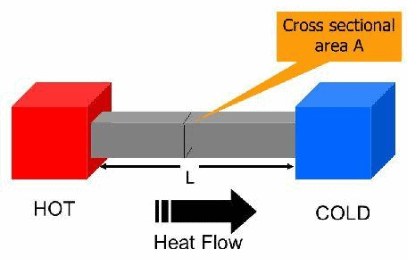Heat Flow rate depends on A/L

When we say that a body is heated it means that its molecules begin to move with greater kinetic energy.

S.I. unit of heat energy is joule (J). Another common unit of heat energy is calorie (cal).

1.1 Mechanical Equivalent of Heat:

In early days heat was not recognized as a form of energy. Heat was supposed to be something needed to raise the temperature of a body or to change its phase. Calorie was defined as the unit of heat.

A number of experiments were performed to show that the temperature may also be increased by doing mechanical work on the system. These experiments established that heat is equivalent to mechanical energy and measured how much mechanical energy is equivalent to a calorie. If mechanical work W produces the same temperature change as heat H, we write,

W = JH

Where J is called mechanical equivalent of heat. J is expressed in joule/calories. The value of J gives how many joules of mechanical work is needed to raise the temperature of 1 g of water by 1°C.

1 calorie: The amount of heat needed to increase the temperature of 1 gm of water from 14.5 to 15.5 °C at one atmospheric pressure is 1 calorie.

1 calorie = 4.186 Joule.

1.2 Specific Heat:

Specific heat of substances is equal to heat gain or released by that substance to raise or fall its temperature by 1°C for a unit mass of substance.

When a body is heated, it gains heat. On the other hand, heat is lost when the body is cooled. The gain or loss of heat is directly proportional to :

(a) the mass of the body DQ µ m

(b) rise or fall of temperature of the body DQ µ DT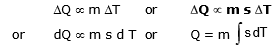where s is a constant and is known as the specific heat of the body s =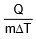S.I. unit of s is joule/kg-kelvin and C.G.S unit is cal/gm °C.

Specific heat of water: s = 4200 J/kg°C = 1000 cal/kg°C = 1 Kcal/kg°C = 1 cal/gm°C.

Specific heat of steam = half of specific heat of water = specific heat of ice

Ex .1 Heat required to increases the temperature of 1 kg water by 20°C

Sol.

heat required = ΔQ = msΔθ
= 1 x 20 = 20 Kcal.
∴ S = 1 cal/gm°C = 1 Kcal/kg°C

Important Points:

(a)  We know, s =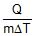, if the substance undergoes the change of state which occurs at constant temperature (DT = 0), the s = Q/0 = ¥. Thus the specific heat of a substance when it melts or boils at constant temperature is infinite.

(b) If the temperature of the substance changes without the transfer of heat (Q = 0) then s == 0. Thus when liquid in the thermos flask is shaken, its temperature increases without the transfer of heat and hence and the specific heat of liquid in the thermos flask is zero.

(c) To raise the temperature of saturated water vapour, heat (Q) is withdrawn. Hence, specific heat of saturated water vapour is negative. (This is for your information only and not in the course)

(d) The slight variation of specific heat of water with temperature is shown in the graph at 1 atmosphere pressure. Its variation is less than 1% over the interval form 0 to 100°C.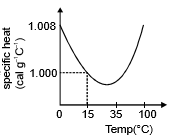1.3 Heat capacity or Thermal capacity:

Heat capacity of a body is defined as the amount of heat required to raise the temperature of that body by 1°C. If `m' is the mass and `s' the specific heat of the body, then

Heat capacity = m s

Units of heat capacity in : CGS system is, cal °C-1SI unit is, JK-1

1.4 Relation between Specific heat and Water equivalent:

It is the amount of water which requires the same amount of heat for the same temperature rise as that of the object

ms AT = mw Sw ΔT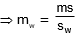In calorie sw = 1
∴ mw = ms
mw is also represented by W
so W = ms

2. Law of Mixture:

When two substances at different temperatures are mixed together, the exchange of heat continues to take place till their temperatures become equal. This temperature is then called final temperature of mixture. Here, Heat taken by one substance = Heat given by another substance.

⇒ m1s1(T1 - Tm) = m2s2(Tm - T2)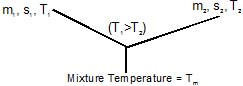Ex. 2 An iron block of mass 2 kg, fall from a height 10 m. After colliding with the ground it loses 25 % energy to surroundings. Then find the temperature rise of the block (Take sp. heat of iron 470 J/kg°C)

Sol.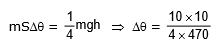Ex. 3 The temperature of equal masses of three different liquids A, B, and C are 10°C 15°C and 20°C respectively. The temperature when A and B are mixed is 13°C and when B and C are mixed, it is 16°C. What will be the temperature when A and C are mixed?

Sol.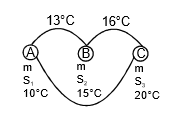when A and B are mixed
mS1 x (13 - 10) = m x S2 x (15 - 13)
3S1 = 2S2    ...(1)
when B and C are mixed
S2 x 1 = S3 x 4    ...(2)
when C and A are mixed
S1(θ - 10) = s3 x (20 - θ)    ,..(3)
by using equation (1), (2) and (3)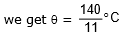Ex. 4 If three different liquid of different masses specific heats and temperature are mixed with each other and then what is the temperature mixture at thermal equilibrium.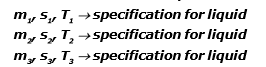Sol. Total heat lost or gain by all substance is equal to zero.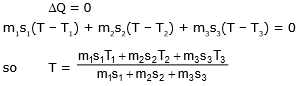Offer running on EduRev: Apply code STAYHOME200 to get INR 200 off on our premium plan EduRev Infinity!

## Physics For JEE

188 videos|346 docs|307 tests

,

,

,

,

,

,

,

,

,

,

,

,

,

,

,

,

,

,

,

,

,

;